Скачать презентацию Advanced Simulation Technologies Conference Smart Product Modeling Dealing

358ea7d317f8b35099c03f565feb60e1.ppt

• Количество слайдов: 47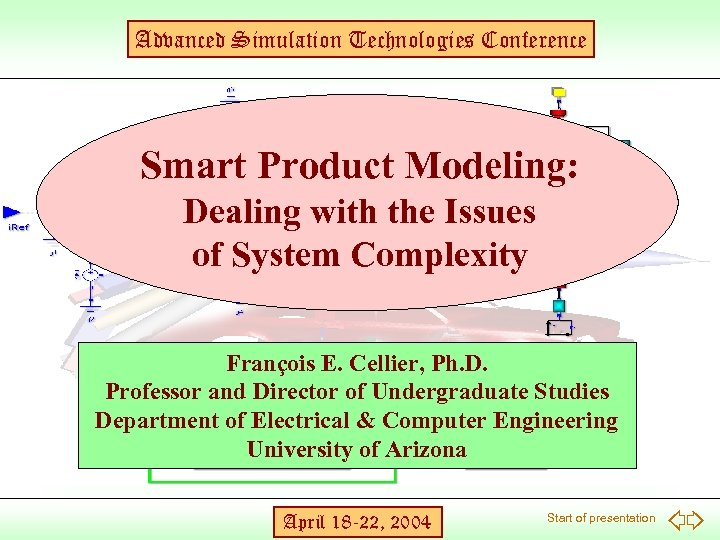Advanced Simulation Technologies Conference Smart Product Modeling: Dealing with the Issues of System Complexity François E. Cellier, Ph. D. Professor and Director of Undergraduate Studies Department of Electrical & Computer Engineering University of Arizona April 18 -22, 2004 Start of presentation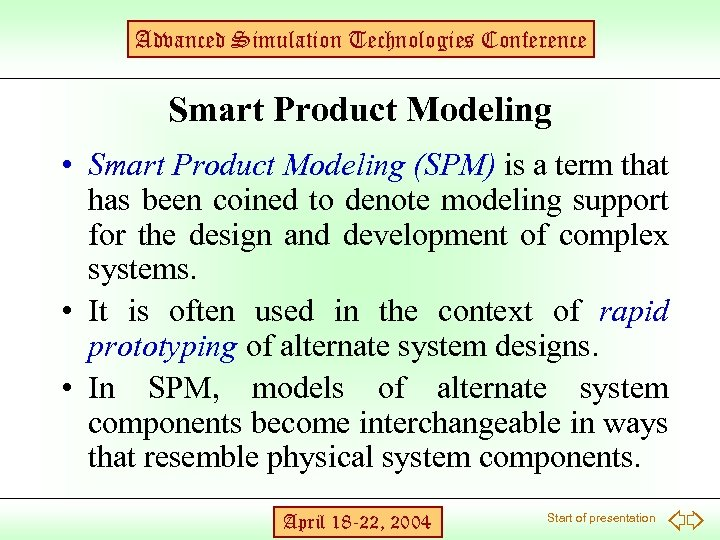Advanced Simulation Technologies Conference Smart Product Modeling • Smart Product Modeling (SPM) is a term that has been coined to denote modeling support for the design and development of complex systems. • It is often used in the context of rapid prototyping of alternate system designs. • In SPM, models of alternate system components become interchangeable in ways that resemble physical system components. April 18 -22, 2004 Start of presentation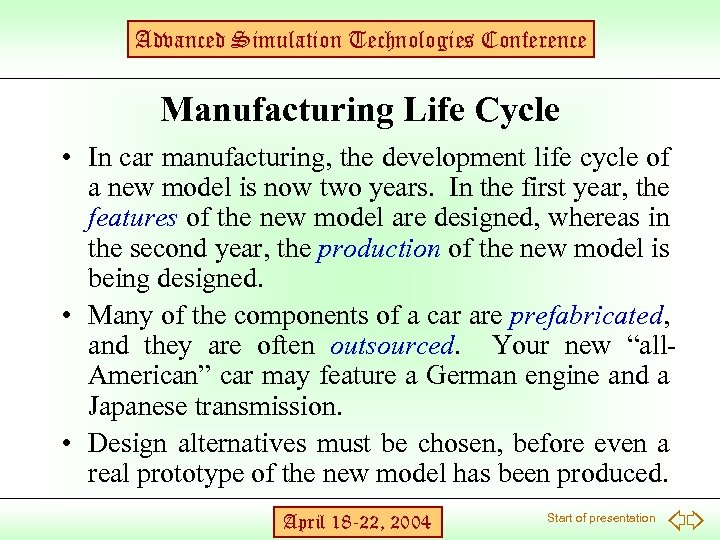Advanced Simulation Technologies Conference Manufacturing Life Cycle • In car manufacturing, the development life cycle of a new model is now two years. In the first year, the features of the new model are designed, whereas in the second year, the production of the new model is being designed. • Many of the components of a car are prefabricated, and they are often outsourced. Your new “all. American” car may feature a German engine and a Japanese transmission. • Design alternatives must be chosen, before even a real prototype of the new model has been produced. April 18 -22, 2004 Start of presentation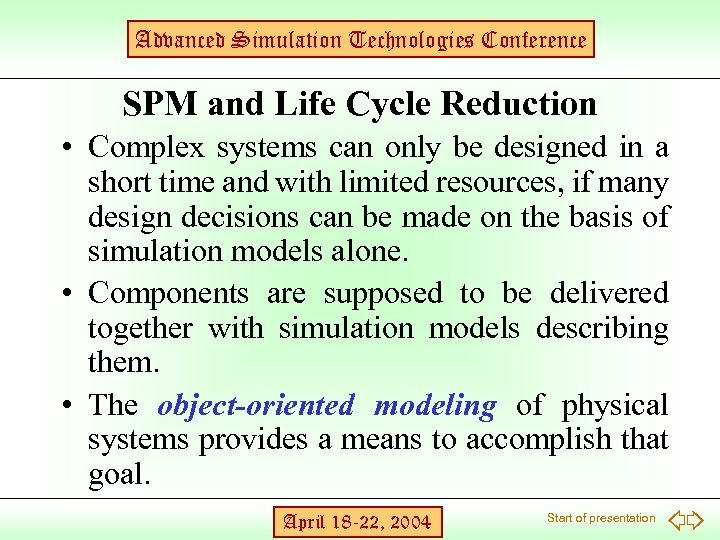Advanced Simulation Technologies Conference SPM and Life Cycle Reduction • Complex systems can only be designed in a short time and with limited resources, if many design decisions can be made on the basis of simulation models alone. • Components are supposed to be delivered together with simulation models describing them. • The object-oriented modeling of physical systems provides a means to accomplish that goal. April 18 -22, 2004 Start of presentation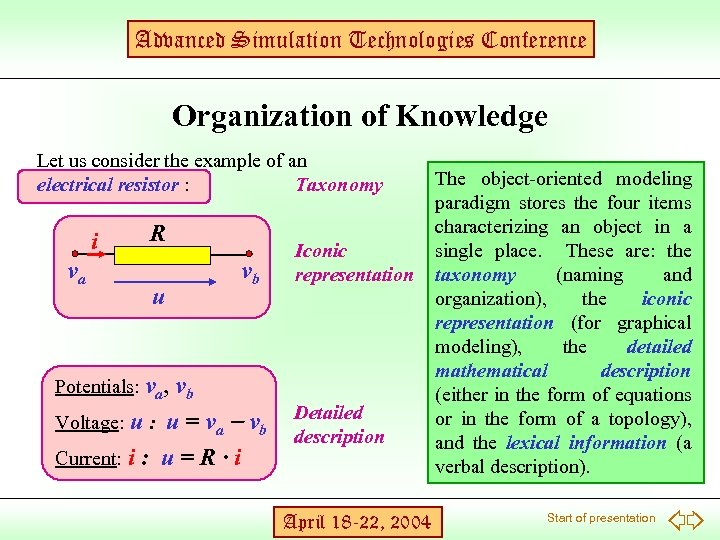Advanced Simulation Technologies Conference Organization of Knowledge Let us consider the example of an electrical resistor : Taxonomy i va R u Potentials: va, vb vb Voltage: u = va - vb Current: i : u = R · i Iconic representation Detailed description April 18 -22, 2004 The object-oriented modeling paradigm stores the four items characterizing an object in a single place. These are: the taxonomy (naming and organization), the iconic representation (for graphical modeling), the detailed mathematical description (either in the form of equations or in the form of a topology), and the lexical information (a verbal description). Start of presentation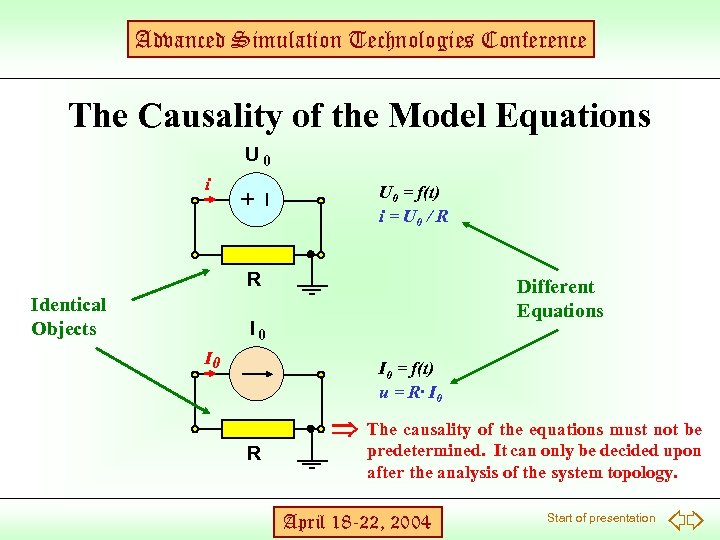Advanced Simulation Technologies Conference The Causality of the Model Equations U 0 i R Identical Objects U 0 = f(t) i = U 0 / R + Different Equations - I 0 I 0 = f(t) u = R· I 0 R The causality of the equations must not be - predetermined. It can only be decided upon after the analysis of the system topology. April 18 -22, 2004 Start of presentation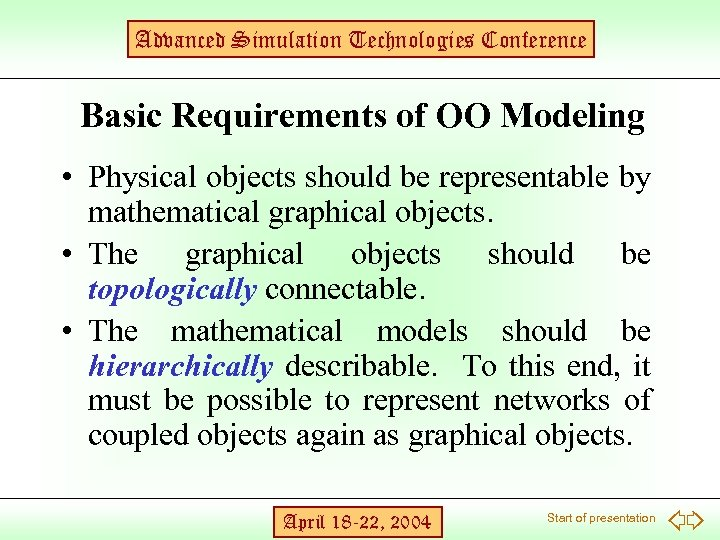Advanced Simulation Technologies Conference Basic Requirements of OO Modeling • Physical objects should be representable by mathematical graphical objects. • The graphical objects should be topologically connectable. • The mathematical models should be hierarchically describable. To this end, it must be possible to represent networks of coupled objects again as graphical objects. April 18 -22, 2004 Start of presentation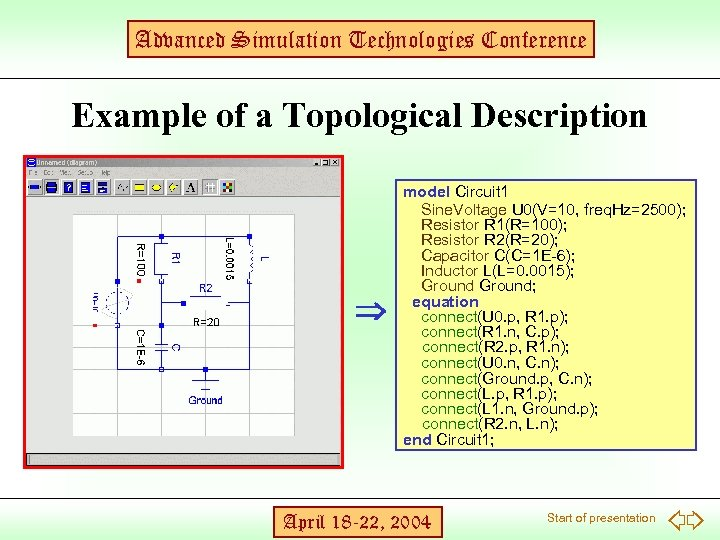Advanced Simulation Technologies Conference Example of a Topological Description model Circuit 1 Sine. Voltage U 0(V=10, freq. Hz=2500); Resistor R 1(R=100); Resistor R 2(R=20); Capacitor C(C=1 E-6); Inductor L(L=0. 0015); Ground; equation connect(U 0. p, R 1. p); connect(R 1. n, C. p); connect(R 2. p, R 1. n); connect(U 0. n, C. n); connect(Ground. p, C. n); connect(L. p, R 1. p); connect(L 1. n, Ground. p); connect(R 2. n, L. n); end Circuit 1; April 18 -22, 2004 Start of presentation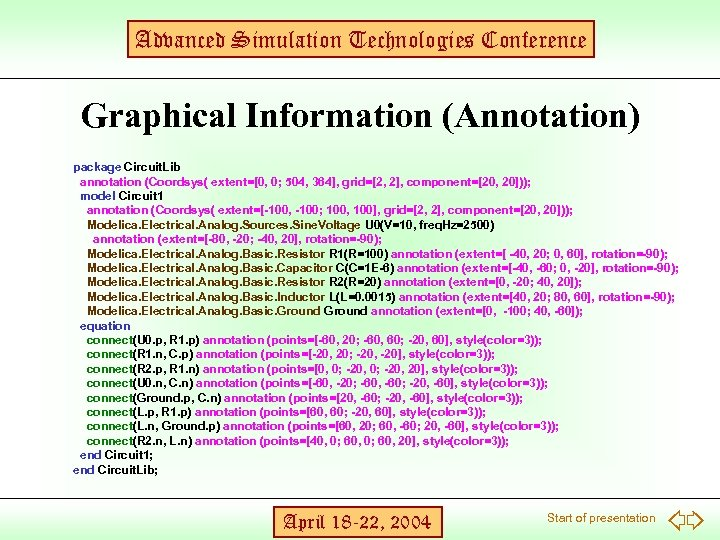Advanced Simulation Technologies Conference Graphical Information (Annotation) package Circuit. Lib annotation (Coordsys( extent=[0, 0; 504, 364], grid=[2, 2], component=[20, 20])); model Circuit 1 annotation (Coordsys( extent=[-100, -100; 100, 100], grid=[2, 2], component=[20, 20])); Modelica. Electrical. Analog. Sources. Sine. Voltage U 0(V=10, freq. Hz=2500) annotation (extent=[-80, -20; -40, 20], rotation=-90); Modelica. Electrical. Analog. Basic. Resistor R 1(R=100) annotation (extent=[ -40, 20; 0, 60], rotation=-90); Modelica. Electrical. Analog. Basic. Capacitor C(C=1 E-6) annotation (extent=[-40, -60; 0, -20], rotation=-90); Modelica. Electrical. Analog. Basic. Resistor R 2(R=20) annotation (extent=[0, -20; 40, 20]); Modelica. Electrical. Analog. Basic. Inductor L(L=0. 0015) annotation (extent=[40, 20; 80, 60], rotation=-90); Modelica. Electrical. Analog. Basic. Ground annotation (extent=[0, -100; 40, -60]); equation connect(U 0. p, R 1. p) annotation (points=[-60, 20; -60, 60; -20, 60], style(color=3)); connect(R 1. n, C. p) annotation (points=[-20, 20; -20, -20], style(color=3)); connect(R 2. p, R 1. n) annotation (points=[0, 0; -20, 20], style(color=3)); connect(U 0. n, C. n) annotation (points=[-60, -20; -60, -60; -20, -60], style(color=3)); connect(Ground. p, C. n) annotation (points=[20, -60; -20, -60], style(color=3)); connect(L. p, R 1. p) annotation (points=[60, 60; -20, 60], style(color=3)); connect(L. n, Ground. p) annotation (points=[60, 20; 60, -60; 20, -60], style(color=3)); connect(R 2. n, L. n) annotation (points=[40, 0; 60, 20], style(color=3)); end Circuit 1; end Circuit. Lib; April 18 -22, 2004 Start of presentation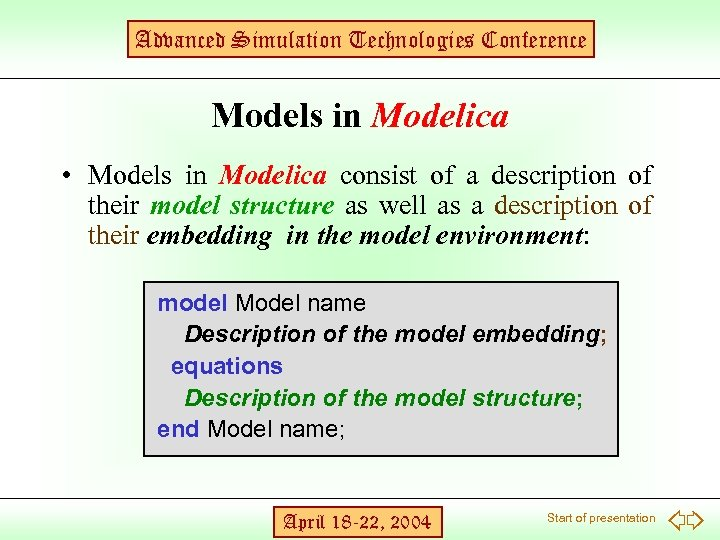Advanced Simulation Technologies Conference Models in Modelica • Models in Modelica consist of a description of their model structure as well as a description of their embedding in the model environment: model Model name Description of the model embedding; equations Description of the model structure; end Model name; April 18 -22, 2004 Start of presentation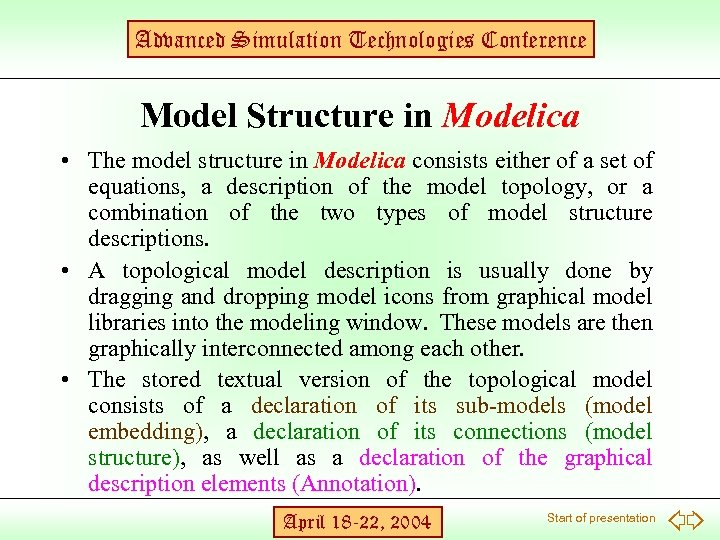Advanced Simulation Technologies Conference Model Structure in Modelica • The model structure in Modelica consists either of a set of equations, a description of the model topology, or a combination of the two types of model structure descriptions. • A topological model description is usually done by dragging and dropping model icons from graphical model libraries into the modeling window. These models are then graphically interconnected among each other. • The stored textual version of the topological model consists of a declaration of its sub-models (model embedding), a declaration of its connections (model structure), as well as a declaration of the graphical description elements (Annotation). April 18 -22, 2004 Start of presentation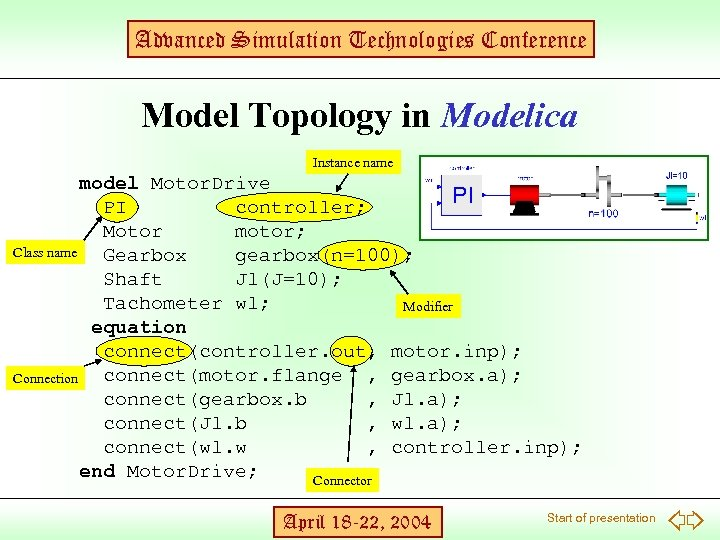Advanced Simulation Technologies Conference Model Topology in Modelica Instance name model Motor. Drive PI controller; Motor motor; Class name Gearbox gearbox(n=100); Shaft Jl(J=10); Tachometer wl; Modifier equation connect(controller. out, motor. inp); connect(motor. flange , gearbox. a); Connection connect(gearbox. b , Jl. a); connect(Jl. b , wl. a); connect(wl. w , controller. inp); end Motor. Drive; Connector April 18 -22, 2004 Start of presentation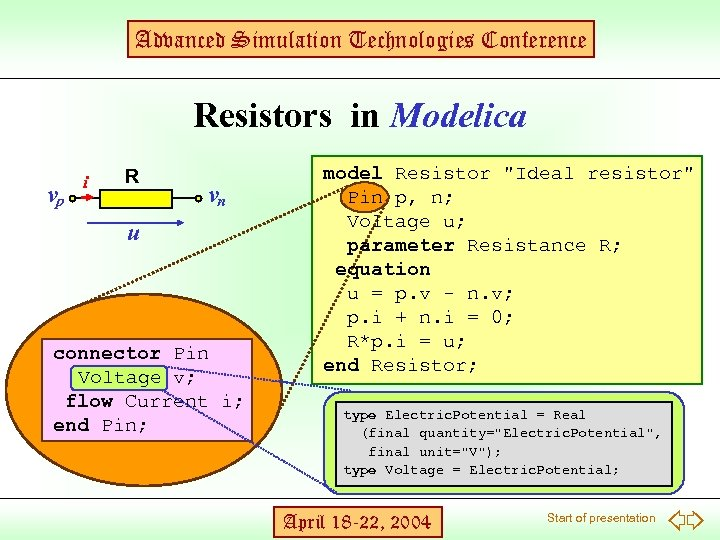Advanced Simulation Technologies Conference Resistors in Modelica vp i R vn u connector Pin Voltage v; flow Current i; end Pin; model Resistor "Ideal resistor" Pin p, n; Voltage u; parameter Resistance R; equation u = p. v - n. v; p. i + n. i = 0; R*p. i = u; end Resistor; type Electric. Potential = Real (final quantity="Electric. Potential", final unit="V"); type Voltage = Electric. Potential; April 18 -22, 2004 Start of presentation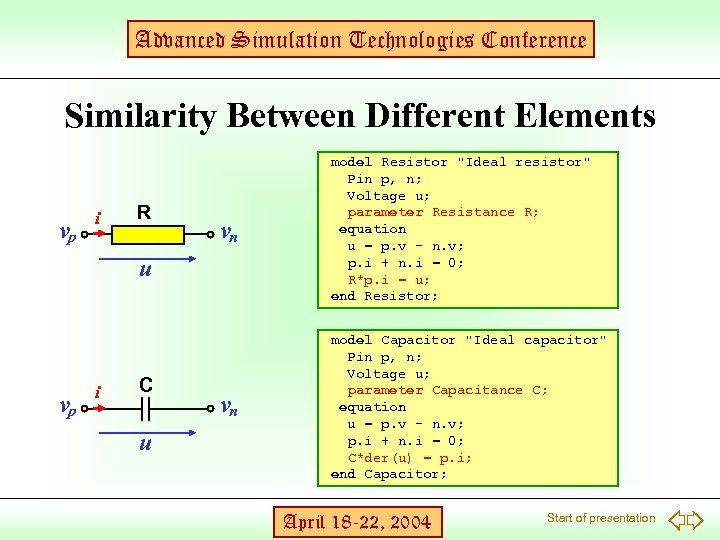Advanced Simulation Technologies Conference Similarity Between Different Elements vp i R vn model Resistor "Ideal resistor" Pin p, n; Voltage u; parameter Resistance R; equation u = p. v - n. v; p. i + n. i = 0; R*p. i = u; end Resistor; vn model Capacitor "Ideal capacitor" Pin p, n; Voltage u; parameter Capacitance C; equation u = p. v - n. v; p. i + n. i = 0; C*der(u) = p. i; end Capacitor; u vp i C u April 18 -22, 2004 Start of presentation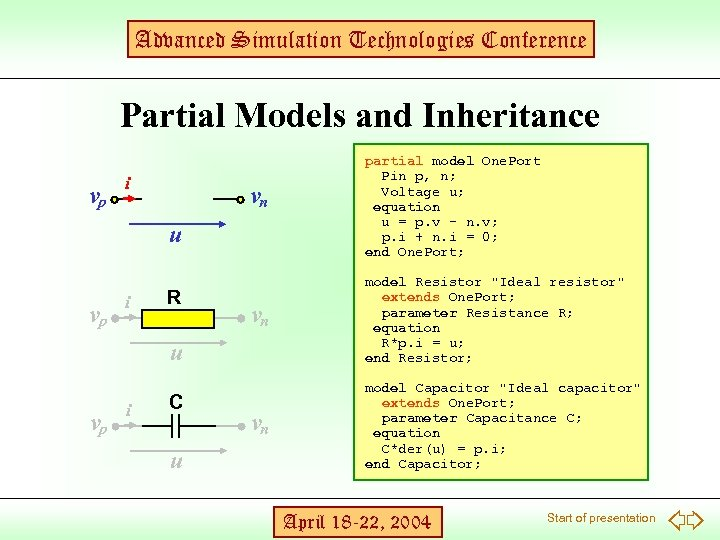Advanced Simulation Technologies Conference Partial Models and Inheritance vp i vn u vp i R vn model Resistor "Ideal resistor" extends One. Port; parameter Resistance R; equation R*p. i = u; end Resistor; vn model Capacitor "Ideal capacitor" extends One. Port; parameter Capacitance C; equation C*der(u) = p. i; end Capacitor; u vp i C u partial model One. Port Pin p, n; Voltage u; equation u = p. v - n. v; p. i + n. i = 0; end One. Port; April 18 -22, 2004 Start of presentation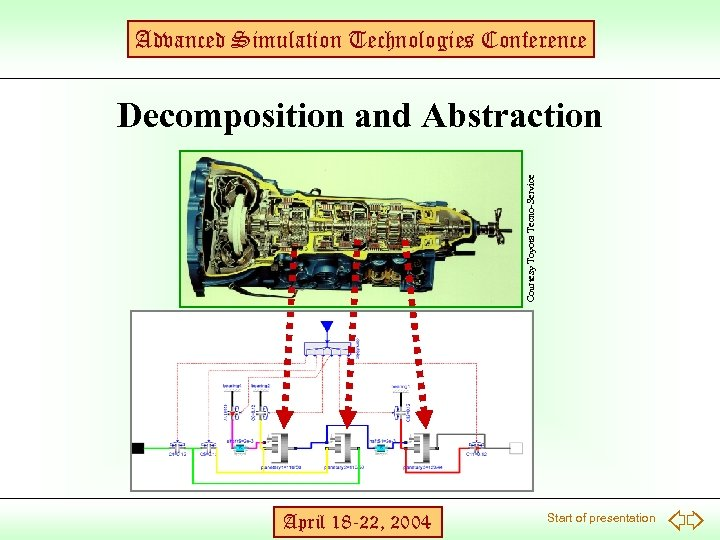Advanced Simulation Technologies Conference Courtesy Toyota Tecno-Service Decomposition and Abstraction April 18 -22, 2004 Start of presentation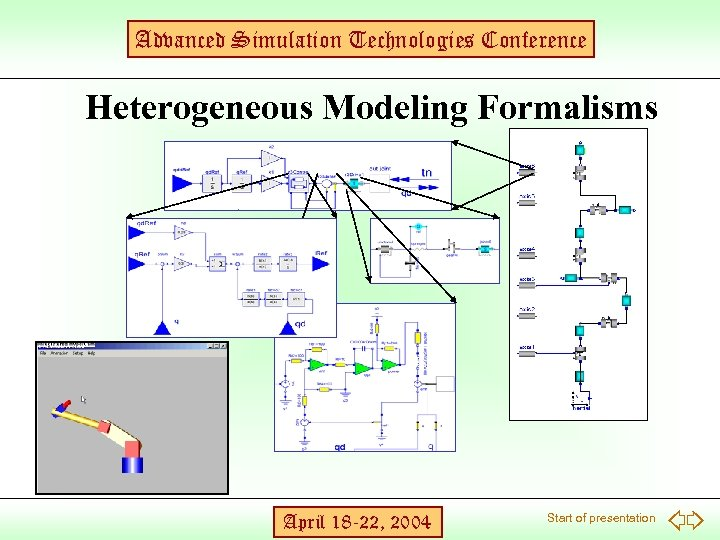Advanced Simulation Technologies Conference Heterogeneous Modeling Formalisms April 18 -22, 2004 Start of presentation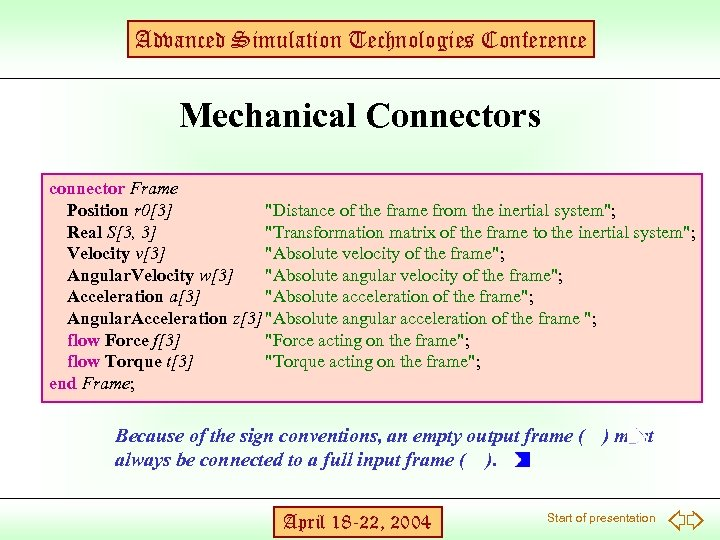Advanced Simulation Technologies Conference Mechanical Connectors connector Frame Position r 0 "Distance of the frame from the inertial system"; Real S[3, 3] "Transformation matrix of the frame to the inertial system"; Velocity v "Absolute velocity of the frame"; Angular. Velocity w "Absolute angular velocity of the frame"; Acceleration a "Absolute acceleration of the frame"; Angular. Acceleration z "Absolute angular acceleration of the frame "; flow Force f "Force acting on the frame"; flow Torque t "Torque acting on the frame"; end Frame; Because of the sign conventions, an empty output frame ( ) must always be connected to a full input frame ( ). April 18 -22, 2004 Start of presentation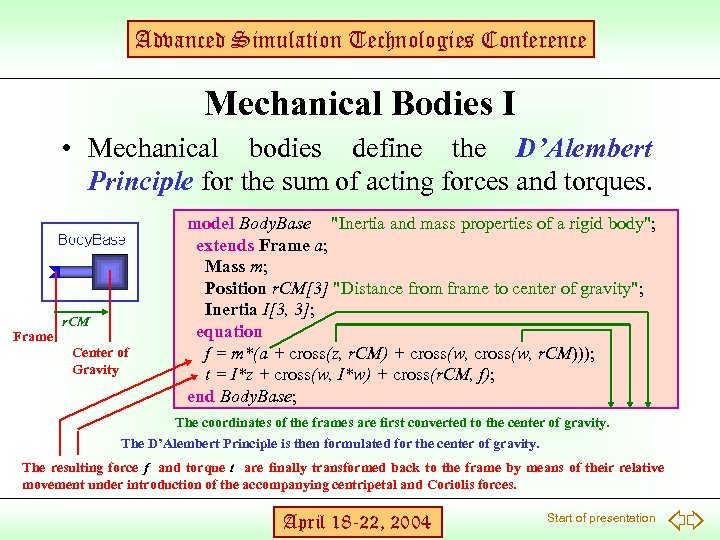Advanced Simulation Technologies Conference Mechanical Bodies I • Mechanical bodies define the D’Alembert Principle for the sum of acting forces and torques. Frame r. CM Center of Gravity model Body. Base "Inertia and mass properties of a rigid body"; extends Frame a; Mass m; Position r. CM "Distance from frame to center of gravity"; Inertia I[3, 3]; equation f = m*(a + cross(z, r. CM) + cross(w, r. CM))); t = I*z + cross(w, I*w) + cross(r. CM, f); end Body. Base; The coordinates of the frames are first converted to the center of gravity. The D’Alembert Principle is then formulated for the center of gravity. The resulting force f and torque t are finally transformed back to the frame by means of their relative movement under introduction of the accompanying centripetal and Coriolis forces. April 18 -22, 2004 Start of presentation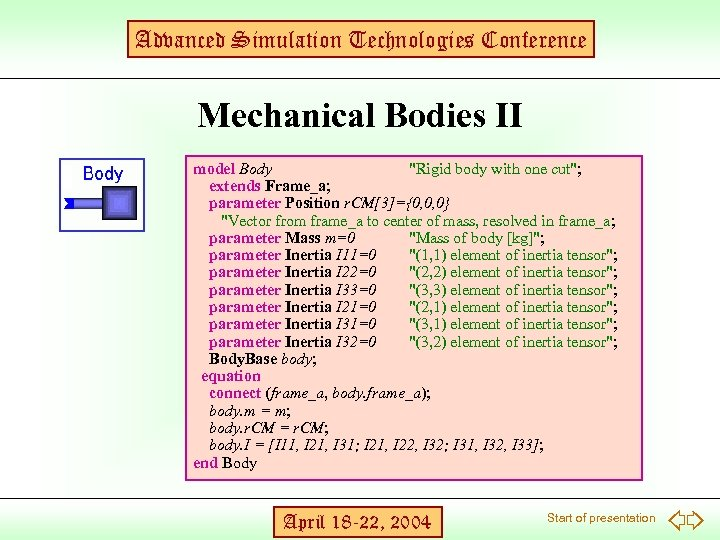Advanced Simulation Technologies Conference Mechanical Bodies II model Body "Rigid body with one cut"; extends Frame_a; parameter Position r. CM={0, 0, 0} "Vector from frame_a to center of mass, resolved in frame_a; parameter Mass m=0 "Mass of body [kg]"; parameter Inertia I 11=0 "(1, 1) element of inertia tensor"; parameter Inertia I 22=0 "(2, 2) element of inertia tensor"; parameter Inertia I 33=0 "(3, 3) element of inertia tensor"; parameter Inertia I 21=0 "(2, 1) element of inertia tensor"; parameter Inertia I 31=0 "(3, 1) element of inertia tensor"; parameter Inertia I 32=0 "(3, 2) element of inertia tensor"; Body. Base body; equation connect (frame_a, body. frame_a); body. m = m; body. r. CM = r. CM; body. I = [I 11, I 21, I 31; I 21, I 22, I 32; I 31, I 32, I 33]; end Body April 18 -22, 2004 Start of presentation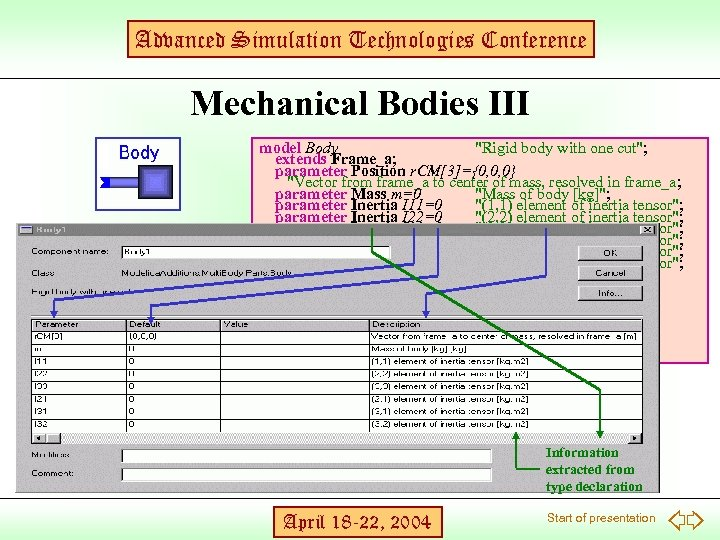Advanced Simulation Technologies Conference Mechanical Bodies III model Body "Rigid body with one cut"; extends Frame_a; parameter Position r. CM={0, 0, 0} "Vector from frame_a to center of mass, resolved in frame_a; parameter Mass m=0 "Mass of body [kg]"; parameter Inertia I 11=0 "(1, 1) element of inertia tensor"; parameter Inertia I 22=0 "(2, 2) element of inertia tensor"; parameter Inertia I 33=0 "(3, 3) element of inertia tensor"; parameter Inertia I 21=0 "(2, 1) element of inertia tensor"; parameter Inertia I 31=0 "(3, 1) element of inertia tensor"; parameter Inertia I 32=0 "(3, 2) element of inertia tensor"; Body. Base body; equation connect (frame_a, body. frame_a); body. m = m; body. r. CM = r. CM; body. I = [I 11, I 21, I 31; I 21, I 22, I 32; I 31, I 32, I 33]; end Body Information extracted from type declaration April 18 -22, 2004 Start of presentation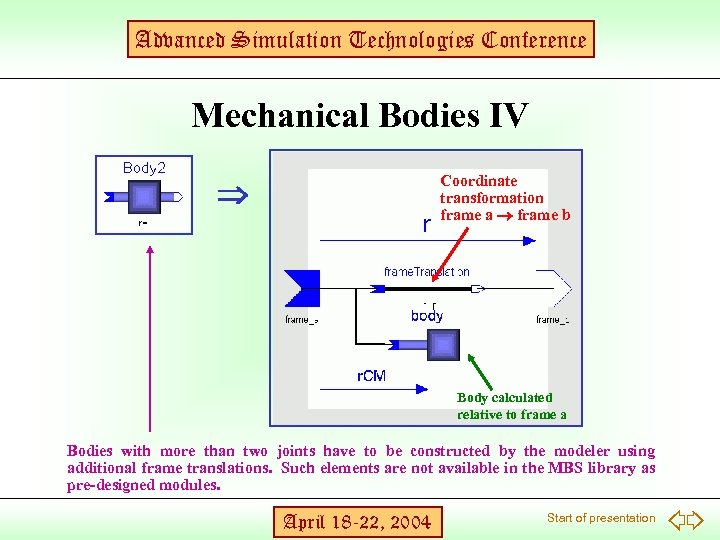Advanced Simulation Technologies Conference Mechanical Bodies IV Coordinate transformation frame a frame b Body calculated relative to frame a Bodies with more than two joints have to be constructed by the modeler using additional frame translations. Such elements are not available in the MBS library as pre-designed modules. April 18 -22, 2004 Start of presentation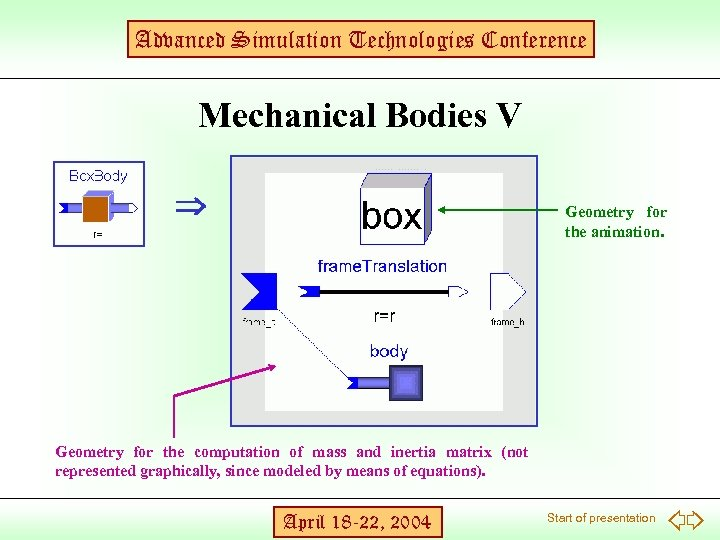Advanced Simulation Technologies Conference Mechanical Bodies V Geometry for the animation. Geometry for the computation of mass and inertia matrix (not represented graphically, since modeled by means of equations). April 18 -22, 2004 Start of presentation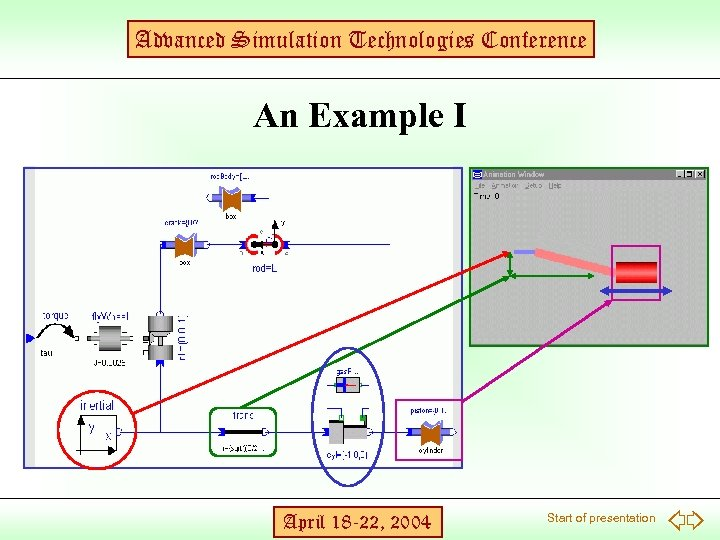Advanced Simulation Technologies Conference An Example I April 18 -22, 2004 Start of presentation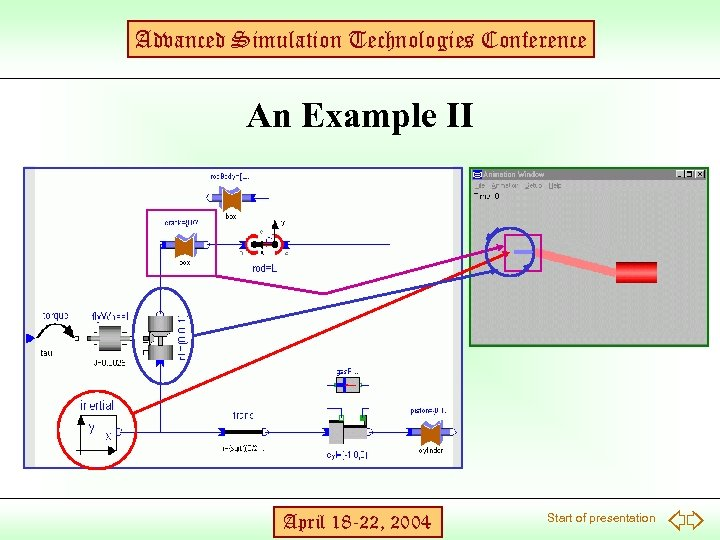Advanced Simulation Technologies Conference An Example II April 18 -22, 2004 Start of presentation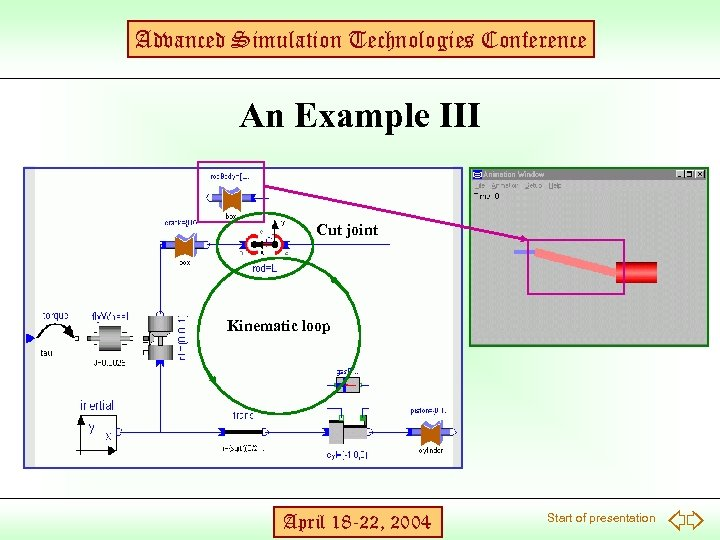Advanced Simulation Technologies Conference An Example III Cut joint Kinematic loop April 18 -22, 2004 Start of presentation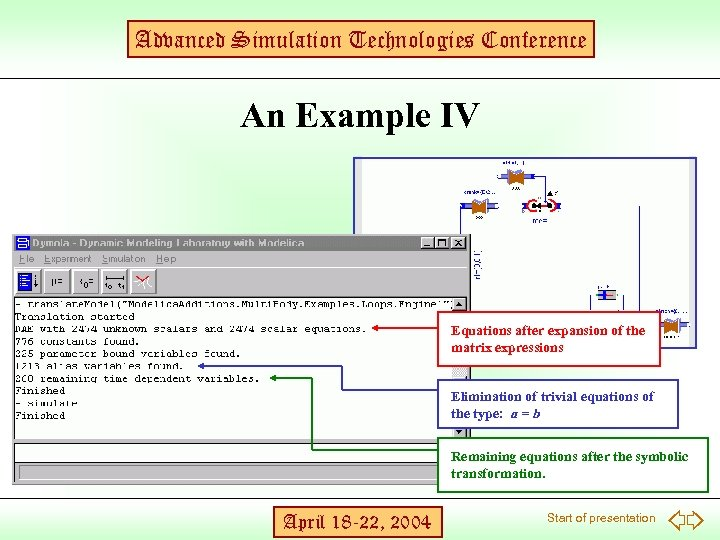Advanced Simulation Technologies Conference An Example IV Equations after expansion of the matrix expressions Elimination of trivial equations of the type: a = b Remaining equations after the symbolic transformation. April 18 -22, 2004 Start of presentation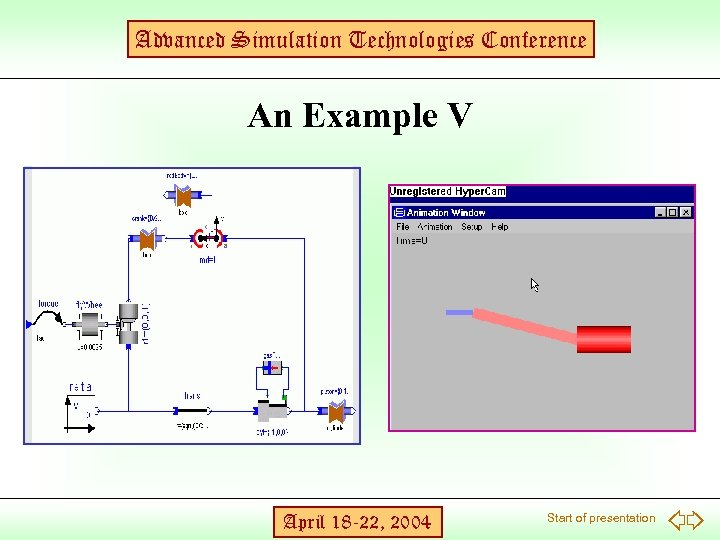Advanced Simulation Technologies Conference An Example V April 18 -22, 2004 Start of presentation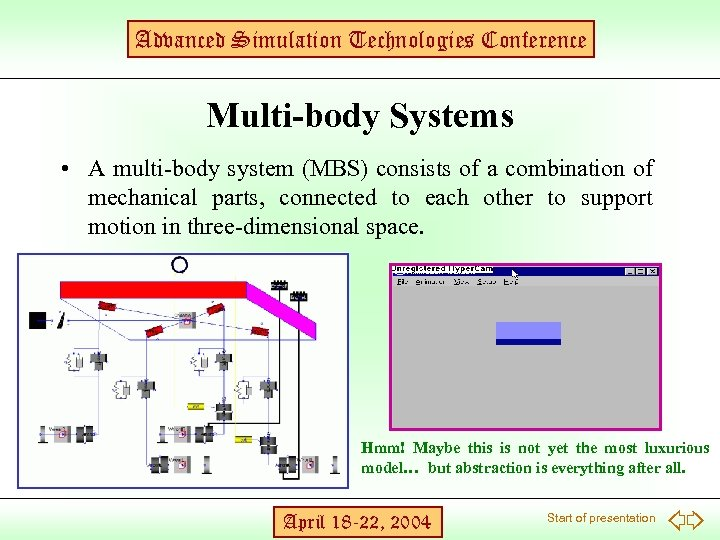Advanced Simulation Technologies Conference Multi-body Systems • A multi-body system (MBS) consists of a combination of mechanical parts, connected to each other to support motion in three-dimensional space. Hmm! Maybe this is not yet the most luxurious model… but abstraction is everything after all. April 18 -22, 2004 Start of presentation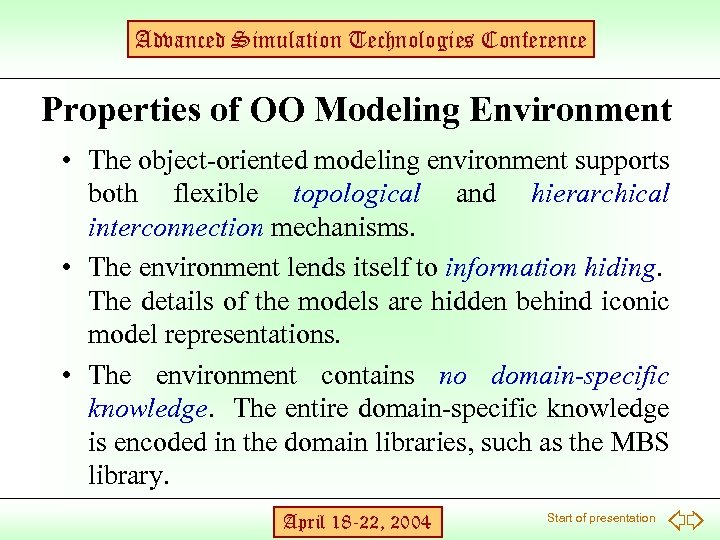Advanced Simulation Technologies Conference Properties of OO Modeling Environment • The object-oriented modeling environment supports both flexible topological and hierarchical interconnection mechanisms. • The environment lends itself to information hiding. The details of the models are hidden behind iconic model representations. • The environment contains no domain-specific knowledge. The entire domain-specific knowledge is encoded in the domain libraries, such as the MBS library. April 18 -22, 2004 Start of presentation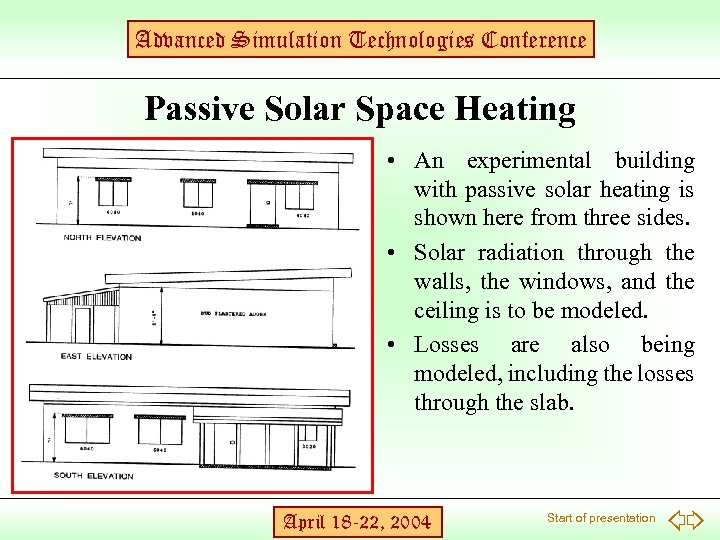Advanced Simulation Technologies Conference Passive Solar Space Heating • An experimental building with passive solar heating is shown here from three sides. • Solar radiation through the walls, the windows, and the ceiling is to be modeled. • Losses are also being modeled, including the losses through the slab. April 18 -22, 2004 Start of presentation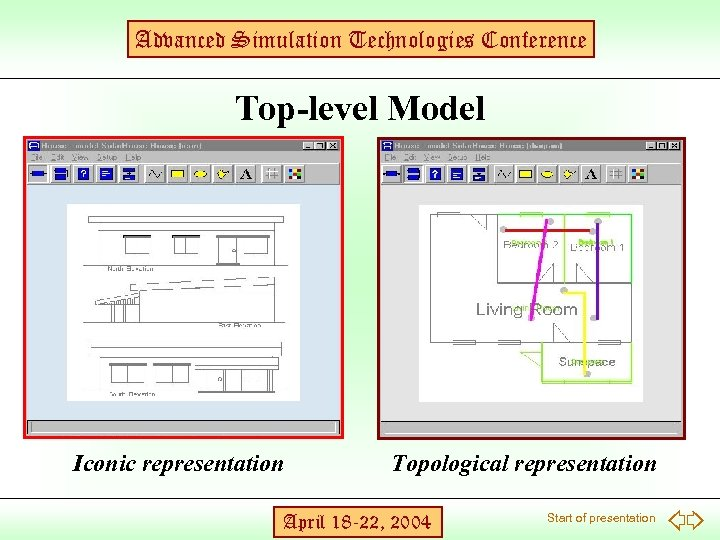Advanced Simulation Technologies Conference Top-level Model Iconic representation Topological representation April 18 -22, 2004 Start of presentation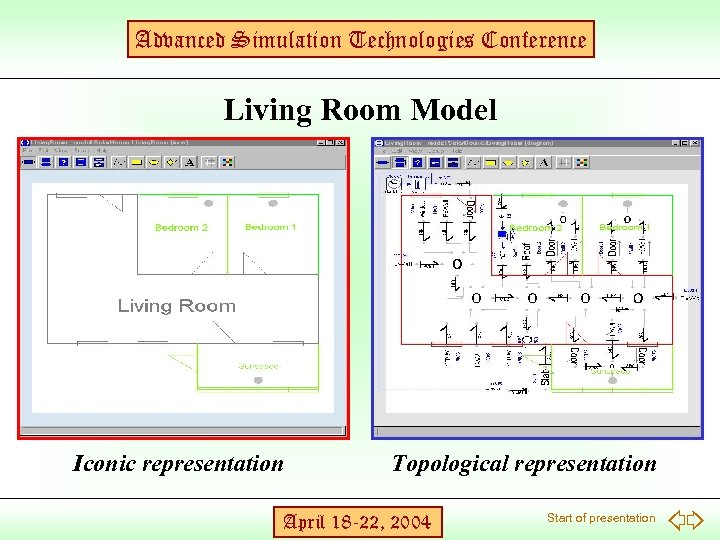Advanced Simulation Technologies Conference Living Room Model Iconic representation Topological representation April 18 -22, 2004 Start of presentation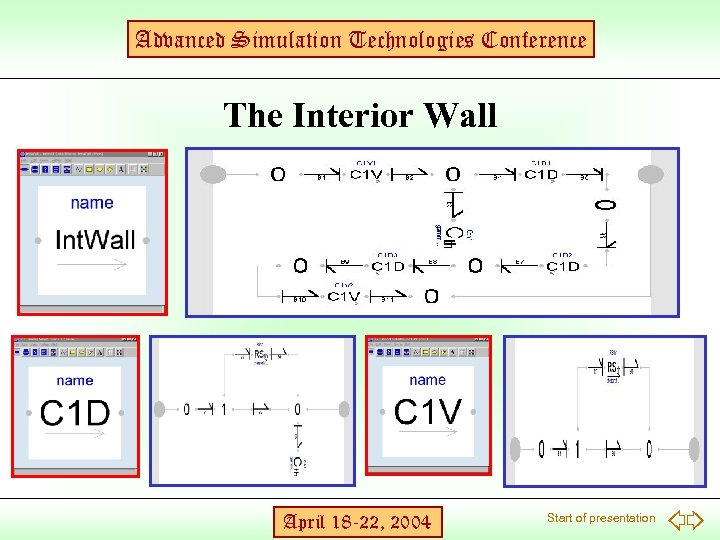Advanced Simulation Technologies Conference The Interior Wall April 18 -22, 2004 Start of presentation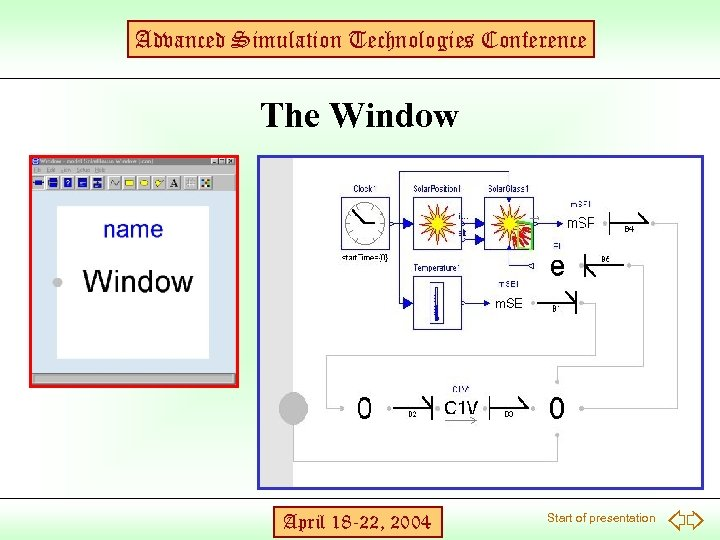Advanced Simulation Technologies Conference The Window April 18 -22, 2004 Start of presentation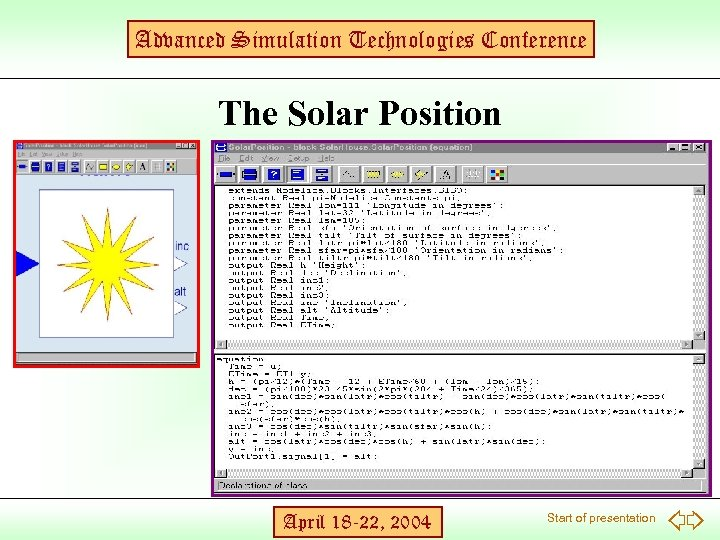Advanced Simulation Technologies Conference The Solar Position April 18 -22, 2004 Start of presentation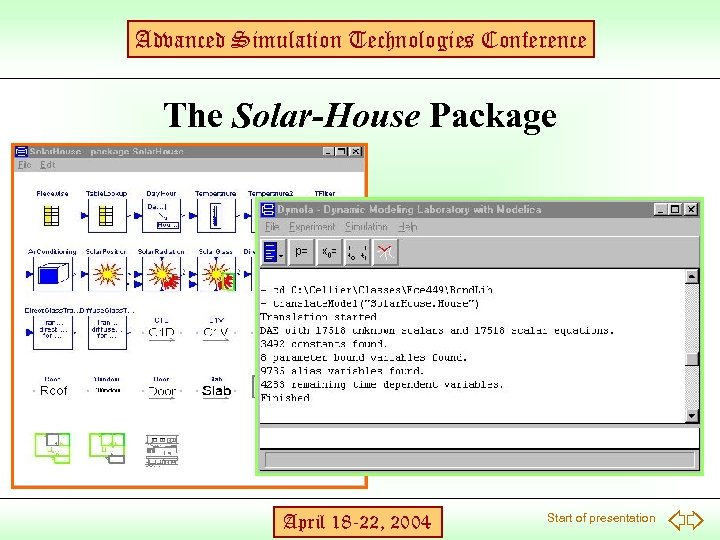Advanced Simulation Technologies Conference The Solar-House Package April 18 -22, 2004 Start of presentation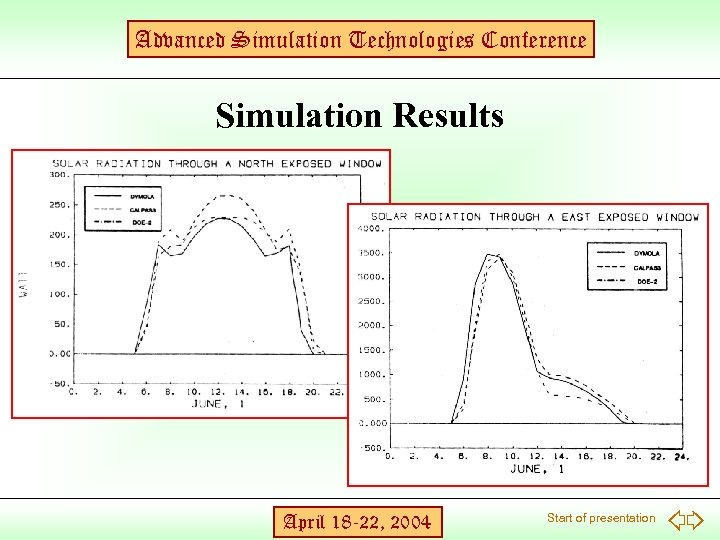Advanced Simulation Technologies Conference Simulation Results April 18 -22, 2004 Start of presentation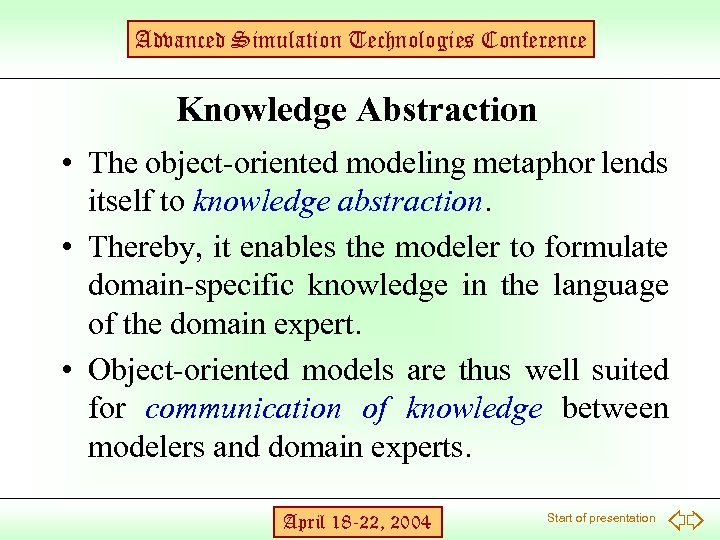Advanced Simulation Technologies Conference Knowledge Abstraction • The object-oriented modeling metaphor lends itself to knowledge abstraction. • Thereby, it enables the modeler to formulate domain-specific knowledge in the language of the domain expert. • Object-oriented models are thus well suited for communication of knowledge between modelers and domain experts. April 18 -22, 2004 Start of presentation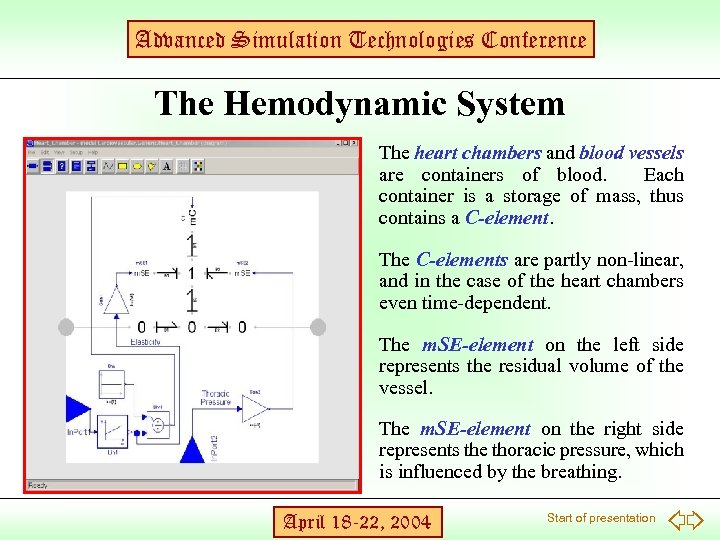Advanced Simulation Technologies Conference The Hemodynamic System The heart chambers and blood vessels are containers of blood. Each container is a storage of mass, thus contains a C-element. The C-elements are partly non-linear, and in the case of the heart chambers even time-dependent. The m. SE-element on the left side represents the residual volume of the vessel. The m. SE-element on the right side represents the thoracic pressure, which is influenced by the breathing. April 18 -22, 2004 Start of presentation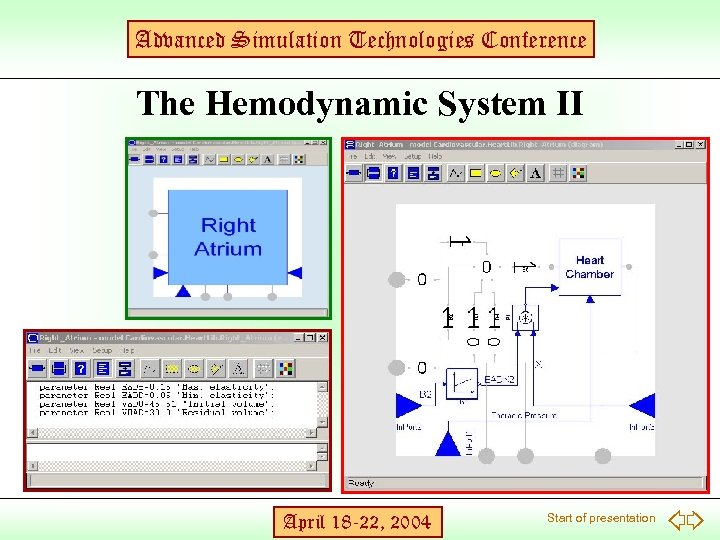Advanced Simulation Technologies Conference The Hemodynamic System II April 18 -22, 2004 Start of presentation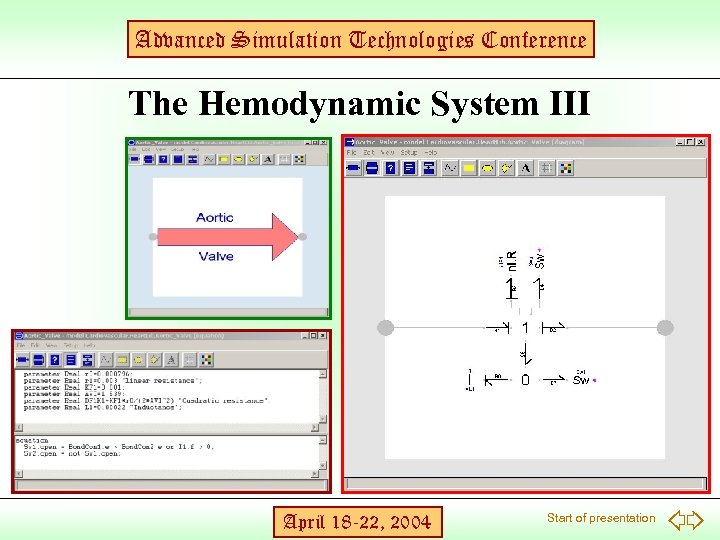Advanced Simulation Technologies Conference The Hemodynamic System III April 18 -22, 2004 Start of presentation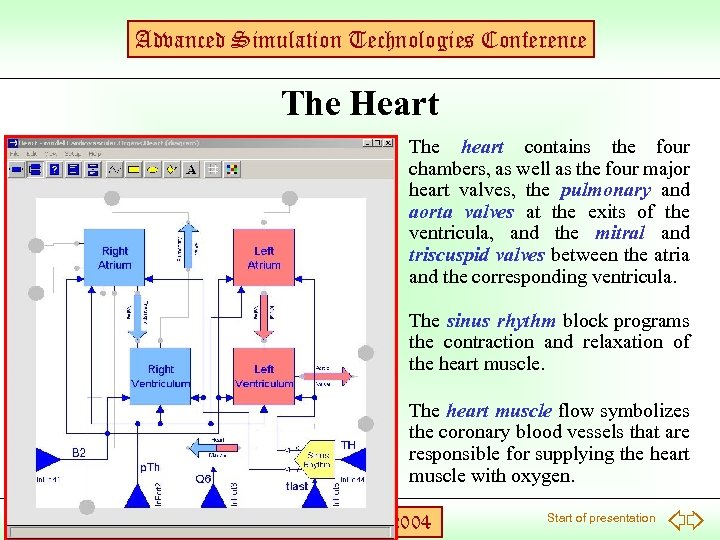Advanced Simulation Technologies Conference The Heart The heart contains the four chambers, as well as the four major heart valves, the pulmonary and aorta valves at the exits of the ventricula, and the mitral and triscuspid valves between the atria and the corresponding ventricula. The sinus rhythm block programs the contraction and relaxation of the heart muscle. The heart muscle flow symbolizes the coronary blood vessels that are responsible for supplying the heart muscle with oxygen. April 18 -22, 2004 Start of presentation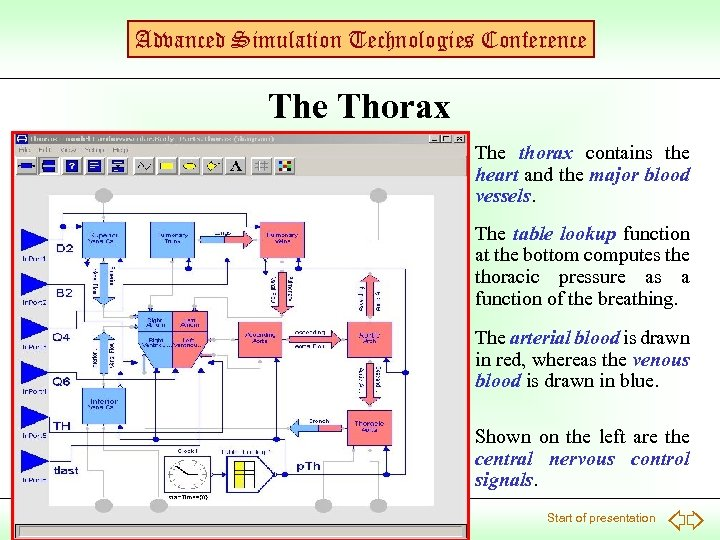Advanced Simulation Technologies Conference Thorax The thorax contains the heart and the major blood vessels. The table lookup function at the bottom computes the thoracic pressure as a function of the breathing. The arterial blood is drawn in red, whereas the venous blood is drawn in blue. Shown on the left are the central nervous control signals. April 18 -22, 2004 Start of presentation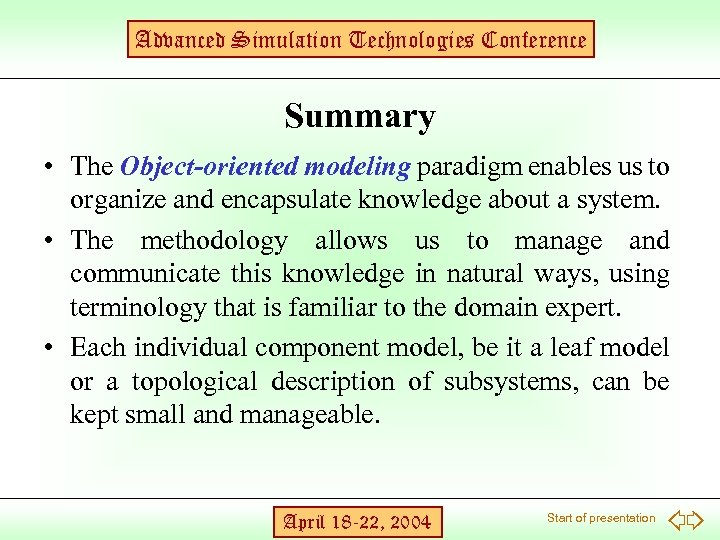Advanced Simulation Technologies Conference Summary • The Object-oriented modeling paradigm enables us to organize and encapsulate knowledge about a system. • The methodology allows us to manage and communicate this knowledge in natural ways, using terminology that is familiar to the domain expert. • Each individual component model, be it a leaf model or a topological description of subsystems, can be kept small and manageable. April 18 -22, 2004 Start of presentation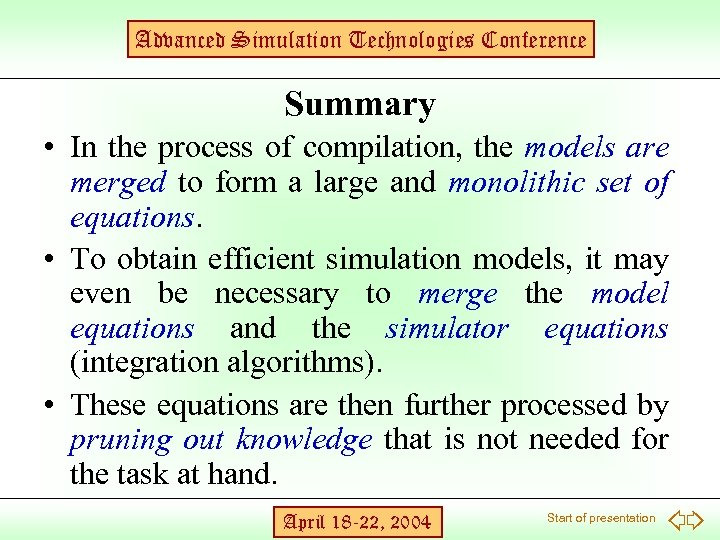Advanced Simulation Technologies Conference Summary • In the process of compilation, the models are merged to form a large and monolithic set of equations. • To obtain efficient simulation models, it may even be necessary to merge the model equations and the simulator equations (integration algorithms). • These equations are then further processed by pruning out knowledge that is not needed for the task at hand. April 18 -22, 2004 Start of presentation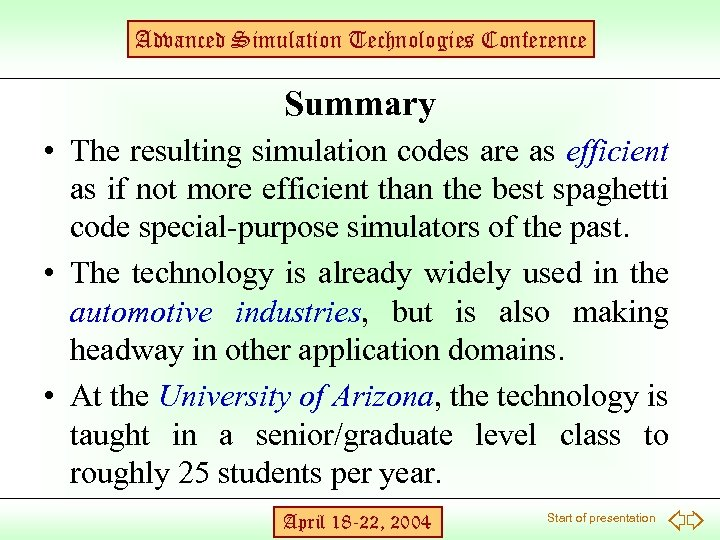Advanced Simulation Technologies Conference Summary • The resulting simulation codes are as efficient as if not more efficient than the best spaghetti code special-purpose simulators of the past. • The technology is already widely used in the automotive industries, but is also making headway in other application domains. • At the University of Arizona, the technology is taught in a senior/graduate level class to roughly 25 students per year. April 18 -22, 2004 Start of presentation